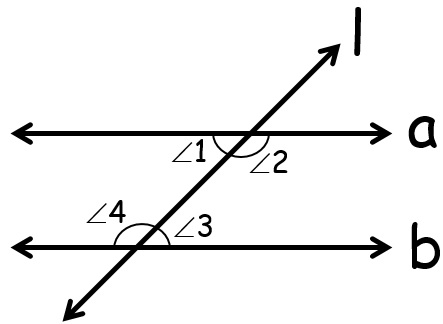# vs.eyeandcontacts.com

## Chapter 5 Introduction to Euclid's Geometry Exercise 5.2

Question 1: How would you rewrite Euclid’s fifth postulate so that it would be easier to understand?
We can rewrite Euclid’s fifth postulate as ‘two distinct intersecting lines cannot be parallel to the same line’.

Question 2: Does Euclid’s fifth postulate imply the existence of parallel lines? Explain.
Yes, Euclid’s fifth postulate does imply the existence of parallel lines.

If the sum of the interior angles is equal to the sum of the right angles, then the two lines will not meet each other at any given point, hence making them parallel to each other.From the figure, ∠1 + ∠4 = 180°
or, ∠3 + ∠4 = 180°

Therefore, the lines a and b never meet, i.e, they are parallel.